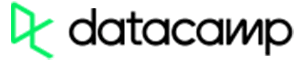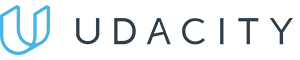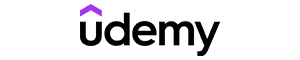Code has been added to clipboard!

# Guide on the Python Map Function for Applying Functions to Sequences

Published Nov 25, 2019
Updated Nov 25, 2019

TL;DR – The Python map function is for applying a specified function to every item in an iterable (a list, a tuple, etc.) and showing the results.

## The basic syntax of the Python map() function

The syntax for writing Python `map()` function looks like this:

``map(function, iterable, ...)``

Let's breakdown each element:

• Function: `map()` will apply this function to every item.
• Iterable: the objects that you will map.

Note: iterable is an object in a list or another sequence that can be taken for iteration. Iteration refers to the process of taking every object and applying a function to it. Basically, this is what the Python `map()` function does.

## Using map in Python with different sequences

### List

A list in Python is a collection of objects that are put inside brackets ([ ]). Lists are more flexible than sets since they can have duplicate objects.

To show the `map` result in a list, insert this line at the end of the code.

``print(list(result))``

Here is a Python `map()` example with a list to complete mathematical calculations.

Example
``````def mul(n):
return n * n
numbers = [4, 5, 2, 9]
result = map(mul, numbers)
print(list(result))``````

The output will look like this:

``[16, 25, 4, 81]``

Alternatively, you can use lambda to get the same result if you don't want to define your function first. For example:

Example
``````numbers = (4, 5, 2, 9)
result = map(lambda x: x * x, numbers)
print(list(result))``````

Note: lambda is a keyword for building anonymous functions. They help you avoid repetitive code when you need to use the same function multiple times in your program. Additionally, lambda is frequently used for small functions that you do not want to define separately.Pros
• Easy to use with a learn-by-doing approach
• Offers quality content
• Gamified in-browser coding experience
• The price matches the quality
• Suitable for learners ranging from beginner to advanced
Main Features
• Free certificates of completion
• Focused on data science skills
• Flexible learning timetablePros
• Simplistic design (no unnecessary information)
• High-quality courses (even the free ones)
• Variety of features
Main Features
• Nanodegree programs
• Suitable for enterprises
• Paid Certificates of completionPros
• Easy to navigate
• No technical issues
• Seems to care about its users
Main Features
• Huge variety of courses
• 30-day refund policy
• Free certificates of completion

### Set

A set is an unordered sequence of items that you have to put inside braces ({ }). In a set, you can't have duplicate items.

Here is how you print the results of `map` when using sets:

``print(set(result))``

Let's say we want to change `'g', 'b', 'e', 'b', 'g'` to uppercase, and eliminate duplicate letters from the sequence. The following code performs this action:

Example
``````def change_upper_case(s):
return str(s).upper()

chars = {'g', 'b', 'e', 'b', 'g'}

result = map(change_upper_case, chars)
print(set(result))``````

This is how you can do the same with lambda:

Example
``````chars = ['g', 'b', 'e', 'b', 'g']
result = list(map(lambda s: str(s).upper(), chars))
print(set(result))``````

These are the remaining items in the result. However, since a set is unordered, the sequence will change every time you run the program.

`` {'E', 'G', 'B',}``

### Tuple

A tuple is a collection of immutable objects. Unlike a list, it can't be changed, and you have to surround it with parentheses [( )].

To get a tuple result, change your print function to look like this.

``print(tuple(result))``

We'll use the same uppercase argument with a tuple. This is how the code would look like:

Example
``````def change_upper_case(s):
return str(s).upper()

char = (5, 'n', 'ghk')

result = map(change_upper_case, char)
print(tuple(result))``````

With the lambda expression, the code looks like this:

Example
``````char = (5, 'n', 'ghk')
result = tuple(map(lambda s: str(s).upper(), char))
print(tuple(result))``````

The output of both examples is as follows:

``('5', 'N', 'GHK')``

## Using multiple map arguments

What if you need to use a `map()` function with more than one argument? Have a look at the following example:

Example
``````def addition(x, y):
return x + y

numbers1 = [5, 6, 2, 8]
numbers2 = [7, 1, 4, 9]
print(list(result))``````

Here is the code in the lambda expression:

Example
``````numbers1 = [5, 6, 2, 8]
numbers2 = [7, 1, 4, 9]

result = map(lambda x, y: x + y, numbers1, numbers2)
print(list(result))``````

Both will return the following result:

``[12, 7, 6, 17]``

You can replace `print(list)` with `print(set)` or `print(tuple)` to change the output.

## Python map: useful tips

• To write the Python `map` function faster, we suggest that you use lambda expressions. However, if you want to reuse the `def` function, it is important to use non-lambda expressions.
• Displaying results in a set can help you delete multiple duplicate items from a large database.

Days
Hours
Minutes
Seconds